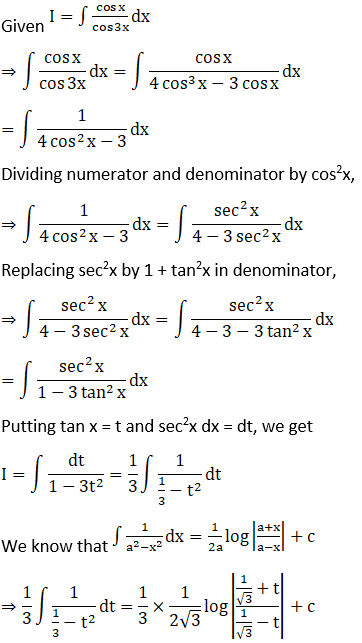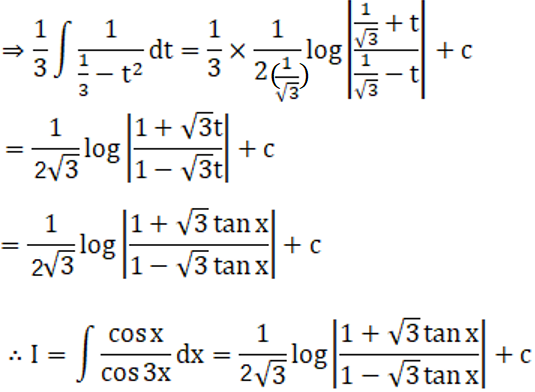# RD Sharma Solutions For Class 12 Maths Exercise 19.22 Chapter 19 Indefinite Integrals

RD Sharma Solutions for Class 12 Maths Exercise 19.22 Chapter 19 Indefinite Integrals is provided here. RD Sharma Solutions are basically designed for CBSE students in accordance with the latest syllabus prescribed by the CBSE Board. The solutions are designed by BYJU’S experts to boost confidence among students in understanding the concepts covered in this chapter. The practice is an essential task to learn and score well in Mathematics.

The PDF of RD Sharma Class 12 Solutions Maths Chapter 19 Indefinite Integrals Exercise 19.22 is provided here. The students are required to go through RD Sharma Solutions thoroughly before the final exams to score well and intensify their problem-solving abilities. Examining the integrals of the form

$$\begin{array}{l}\int \frac{1}{\sqrt{a \sin^{2}x+b \cos^{2}x}}\ dx, \int \frac{1}{a+b \sin^{2}x}\ dx\end{array}$$
is the topic covered under this exercise.

## Download the PDF of RD Sharma Solutions For Class 12 Chapter 19 – Indefinite Integrals Exercise 19.22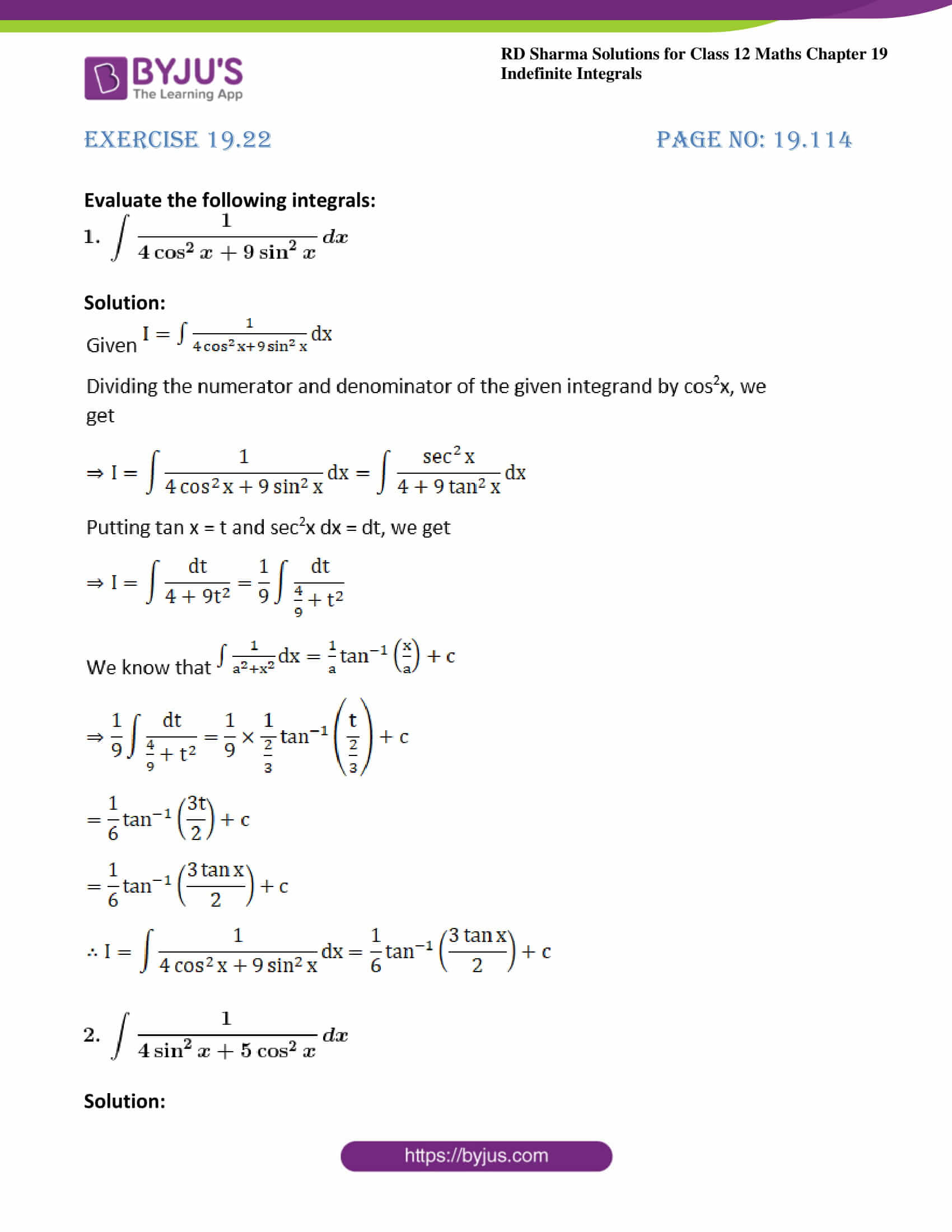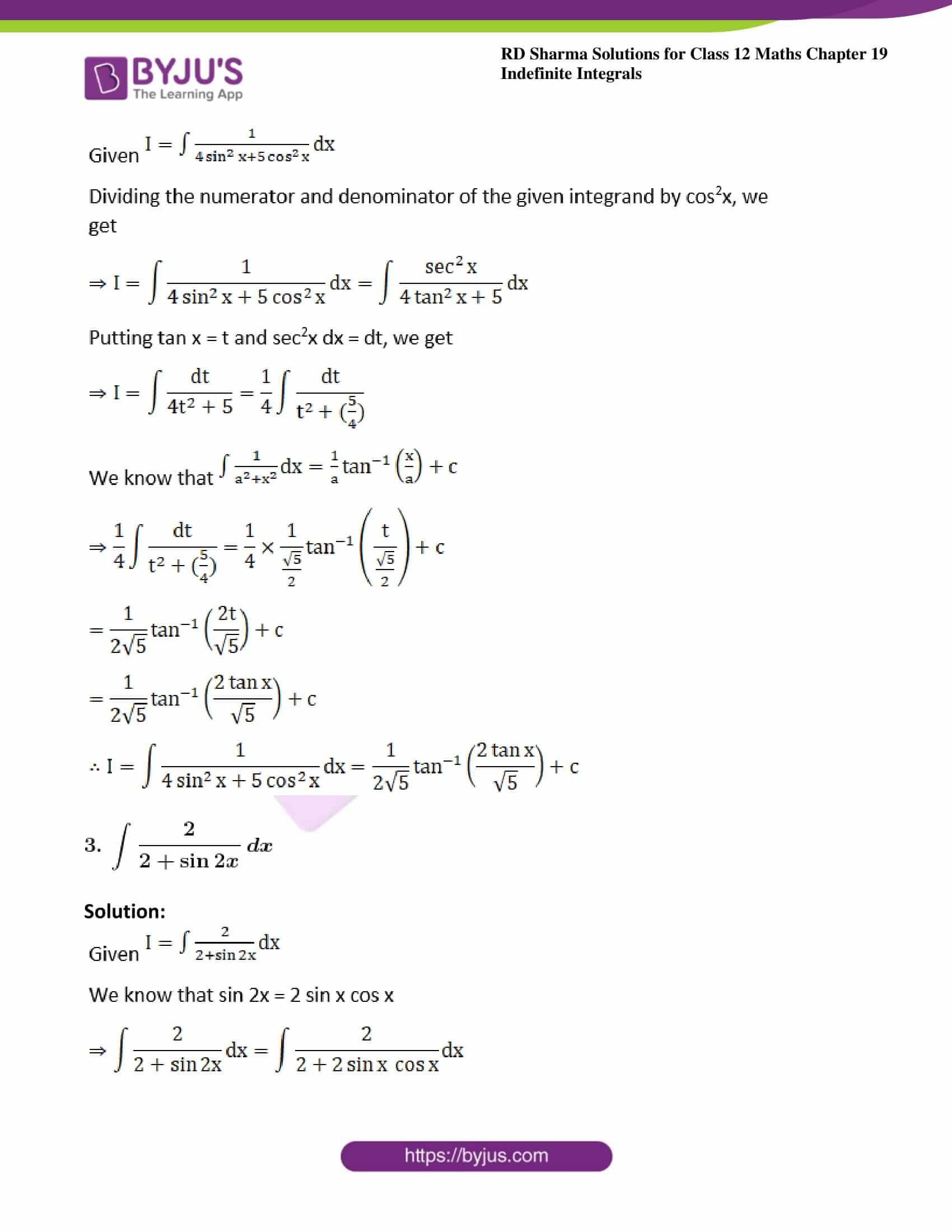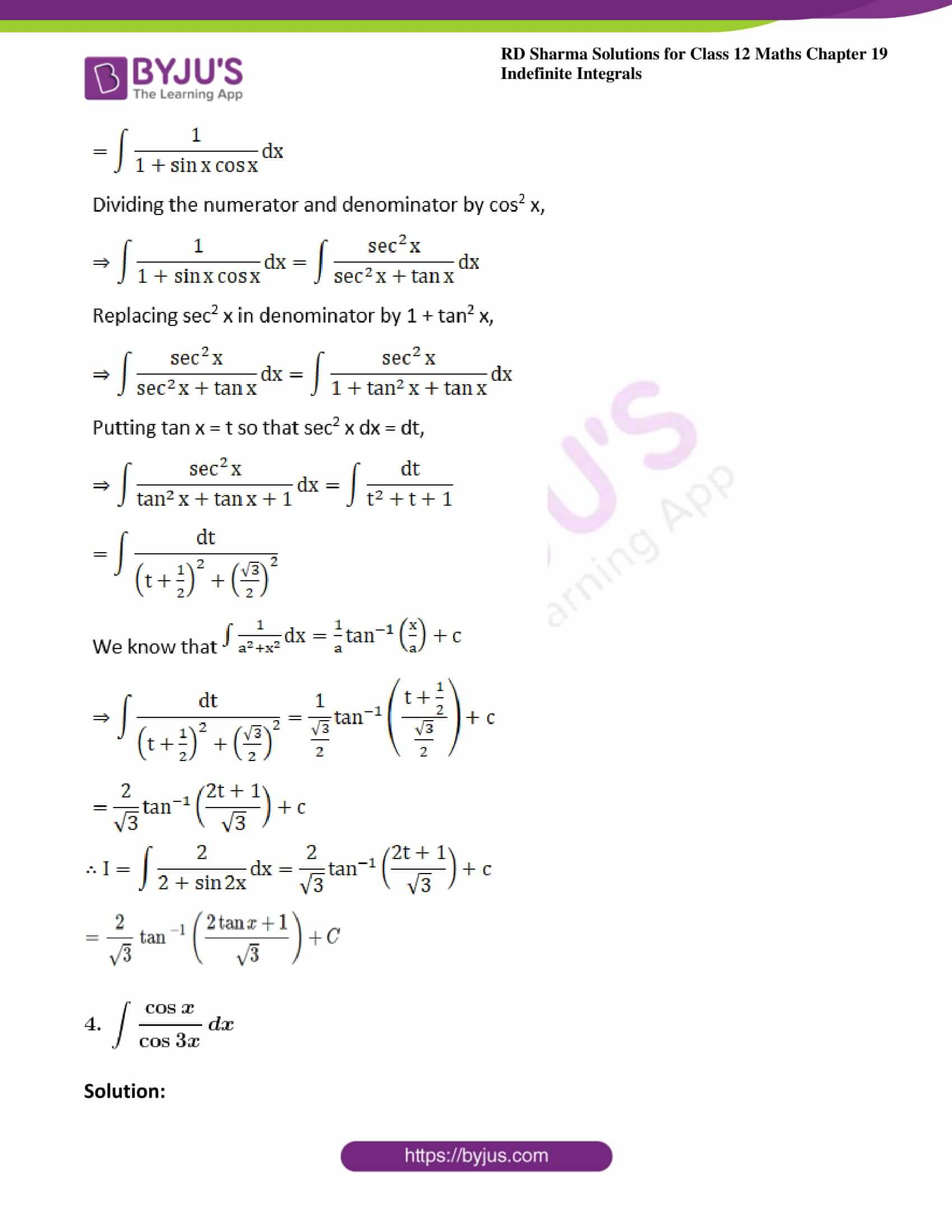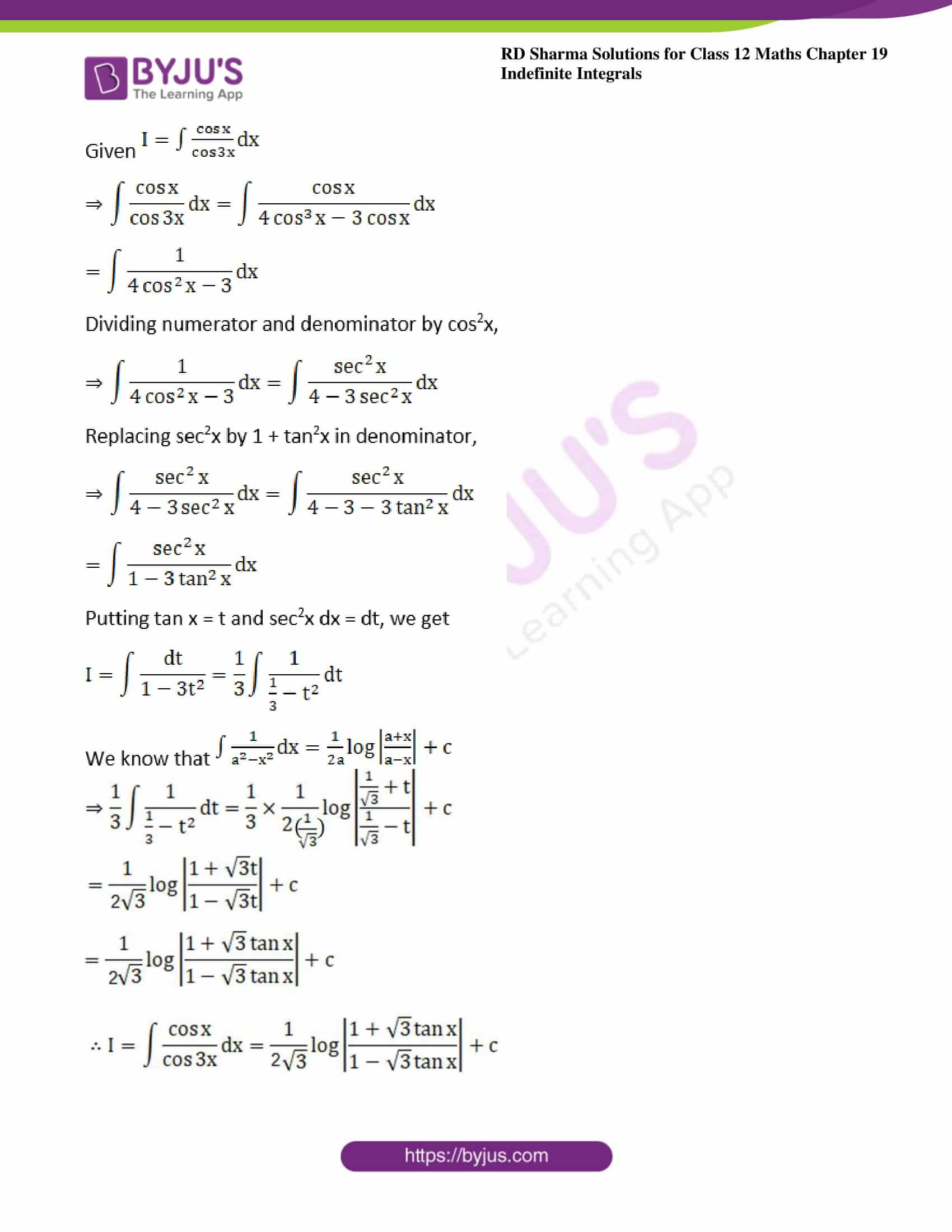### Access other exercises of RD Sharma Solutions For Class 12 Chapter 19 – Indefinite Integrals

Exercise 19.1 Solutions

Exercise 19.2 Solutions

Exercise 19.3 Solutions

Exercise 19.4 Solutions

Exercise 19.5 Solutions

Exercise 19.6 Solutions

Exercise 19.7 Solutions

Exercise 19.8 Solutions

Exercise 19.9 Solutions

Exercise 19.10 Solutions

Exercise 19.11 Solutions

Exercise 19.12 Solutions

Exercise 19.13 Solutions

Exercise 19.14 Solutions

Exercise 19.15 Solutions

Exercise 19.16 Solutions

Exercise 19.17 Solutions

Exercise 19.18 Solutions

Exercise 19.19 Solutions

Exercise 19.20 Solutions

Exercise 19.21 Solutions

Exercise 19.23 Solutions

Exercise 19.24 Solutions

Exercise 19.25 Solutions

Exercise 19.26 Solutions

Exercise 19.27 Solutions

Exercise 19.28 Solutions

Exercise 19.29 Solutions

Exercise 19.30 Solutions

Exercise 19.31 Solutions

Exercise 19.32 Solutions

### Exercise 19.22 Page No: 19.114

Evaluate the following integrals: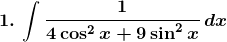Solution: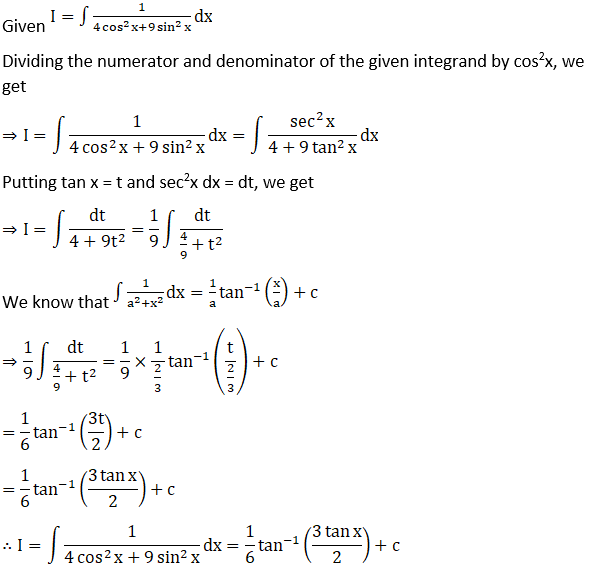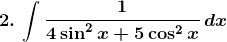Solution: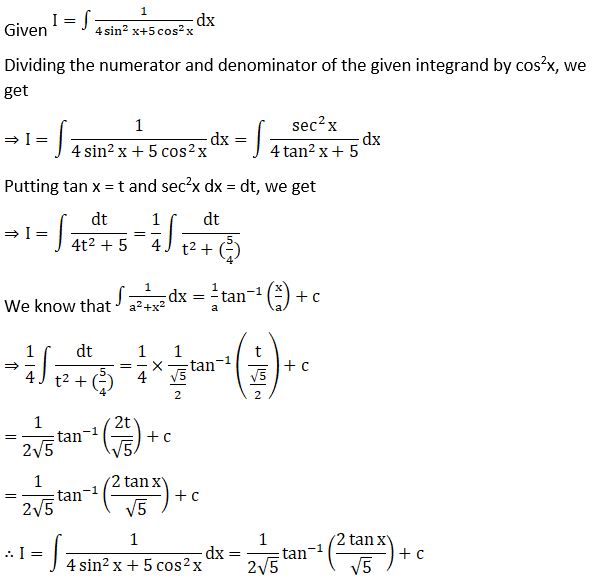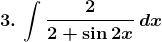Solution: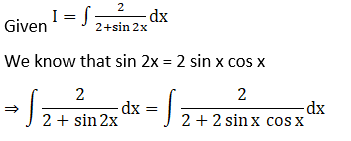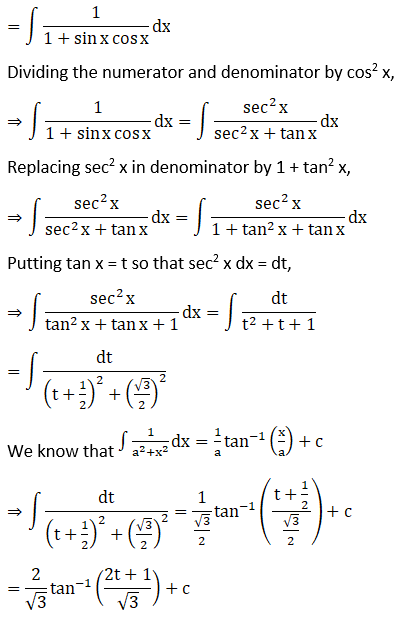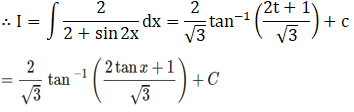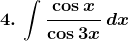Solution: